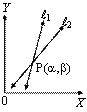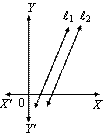# Mathematics

### Chapter : Linear Equation in Two Variable

#### Graph of linear equation ax + by + c = 0 in two variables, where a and b not equal to zero

Let the system of pair of linear equations be

a1x + b1y = c1 ....(1)
a2x + b2y = c2 ....(2)

We know that given two lines in a plane, only one of the following three possibilities can happen -

• The two lines will intersect at one point.
• The two lines are coincident lines.
• The two lines will not intersect, however far they are extended, i.e., they are parallel.Example: The path of highway number 1 is given by the equation x + y = 7 and the highway number 2 is given by the equation 5x + 2y = 20. Represent these equations geometrically.

Solution: We have, x + y = 7
⇒ y = 7 – x ....(1)
In tabular form

 Points A B x 1 4 y 6 3

and 5x + 2y = 20
⇒ y = (20 - 5x)/2 ....(2)
In tabular form

 Points C D x 2 4 y 5 0

Plot the points A (1, 6), B(4, 3) and join them to form a line AB.

Similarly, plot the points C(2, 5). D (4, 0) and join them to get a line CD. Clearly, the two lines intersect at the point C. Now, every point on the line AB gives us a solution of equation (1). Every point on CD gives us a solution of equation (2).

Example: A father tells his daughter, “Seven years ago, I was seven times as old as you were then. Also, three years from now, I shall be three times as old as you will be.” Represent this situation algebraically and graphically.

Solution: Let the present age of father be x-years and that of daughter = y years
Seven years ago father’s age
= (x – 7) years
Seven years ago daughter’s age
= (y – 7) years
According to the problem
(x – 7) = 7(y – 7)
or x – 7y = – 42 ....(1)
After 3 years father’s age = (x + 3) years
After 3 years daughter’s age = (y + 3) years
According to the condition given in the question
x + 3 = 3(y + 3)
or x – 3y = 6 ....(2)

 x 0 7 14 y = (x + 42)/7 6 7 8 Points A B C

x – 7y = –42

x – 3y = 6

 x 6 12 18 y = (x − 6)/3 0 2 4 Points D E F

Plot the points A(0, 6), B(7, 7), C(14, 8) and join them to get a straight line ABC. Similarly plot the points D(6, 0), E(12, 2) and F(18, 4) and join them to get a straight line DEF.